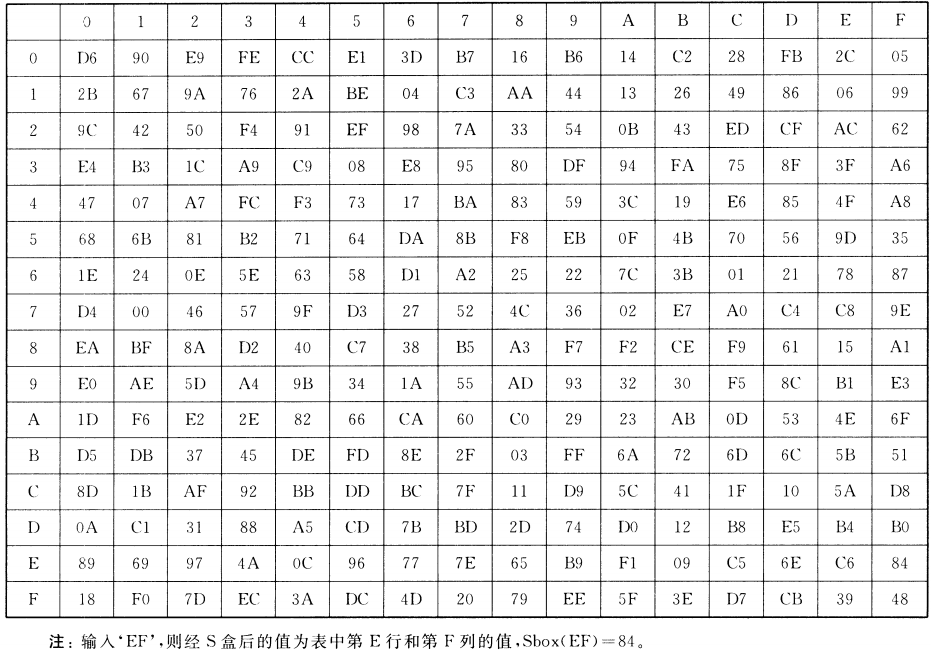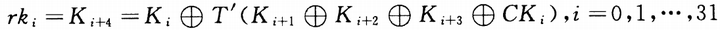# SM4国密算法

## SM4

SM4密码算法是我国自主设计的分组对称密码算法，用于实现数据的加密/解密运算，以保证数据和信息的机密性。

1. 该算法的分组长度为128位（即16字节，4字），密钥长度为128位（即16字节，4字）。
2. 加密算法与密钥扩展算法都采用32轮非线性迭代结构。
3. 密钥扩展算法：将加密密钥变换为轮密钥的运算单元。
4. 数据解密和数据加密的算法结构相同，只是轮密钥的使用顺序相反，解密轮密钥是加密轮密钥的逆序。SM4的分组长度为4字，因此，其输入的是4字明文$(X_0,X_1,X_2,X_3)$，加密后输出4字密文$(Y_0,Y_1,Y_2,Y_3)$。加密过程分为两步，由32次轮迭代和1次反序变换组成。

### 32次轮迭代

#### F函数

$F(X_i,X_{i+1},X_{i+2},X_{i+3},rk_i)=X_i\bigoplus T(X_{i+1}\bigoplus X_{i+2}\bigoplus X_{i+3}\bigoplus rk_i)$$T$​为合成置换。

• 合成置换$T$:

合成置换$T$接受1字的输入$A$，得到1字的输出$C$。它包含非线性变换$\tau$和线性变换$L$，即$C=T(A)=L(\tau(A))$

1. 非线性变换$\tau$

非线性变换接受1字的输入，记为$A=(a_0,a_1,a_2,a_3)$，输出为1字的结果，记为$B=(b_0,b_1,b_2,b_3)$

非线性变换就是对输入参数的每个字节进行S盒变换，得到输出结果。S盒作用和AES中的类似，就是替换字节。2. 线性变换$L$​:

运算公式如下

其中<<<为循环位移

### SM4密钥扩展算法

SM4的密钥为128位(4字)，经过密钥扩展，获得32个1字的轮密钥。记原密钥为$MK=(MK_0,MK_1,MK_2,MK_3)$

#### 迭代方法#### 置换T'​

$L'$是个线性变换函数：

#### $CK_i$的取值

$CK_i$的长为1字，即4字节，记为$CK_i=(ck_{i,0},ck_{i,1},ck_{i,2},ck_{i,3})$。其中$ck_{i,j}=7(4i+j)\quad(mod \ \ 256)$

（SM4那题明天再写咕咕咕）